# JP2012018487A - Node determination program, node determination apparatus, and node determination method - Google Patents

Node determination program, node determination apparatus, and node determination method Download PDF

## Info

Publication number
JP2012018487A
JP2012018487A JP2010154332A JP2010154332A JP2012018487A JP 2012018487 A JP2012018487 A JP 2012018487A JP 2010154332 A JP2010154332 A JP 2010154332A JP 2010154332 A JP2010154332 A JP 2010154332A JP 2012018487 A JP2012018487 A JP 2012018487A
Authority
JP
Japan
Prior art keywords
node
function
data
nodes
step
Prior art date
Legal status (The legal status is an assumption and is not a legal conclusion. Google has not performed a legal analysis and makes no representation as to the accuracy of the status listed.)
Withdrawn
Application number
JP2010154332A
Other languages
Japanese (ja)
Inventor
Yuichi Tsuchimoto

Original Assignee
Fujitsu Ltd

Priority date (The priority date is an assumption and is not a legal conclusion. Google has not performed a legal analysis and makes no representation as to the accuracy of the date listed.)
Filing date
Publication date
Application filed by Fujitsu Ltd, 富士通株式会社 filed Critical Fujitsu Ltd
Priority to JP2010154332A priority Critical patent/JP2012018487A/en
Publication of JP2012018487A publication Critical patent/JP2012018487A/en
Application status is Withdrawn legal-status Critical

• 230000003247 decreasing Effects 0 abstract 1
• 230000001965 increased Effects 0 abstract 1

## Images

•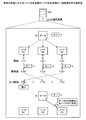•••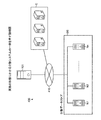••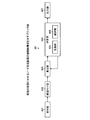•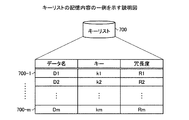•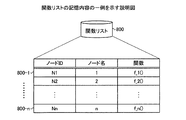•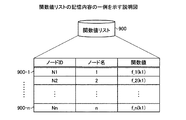•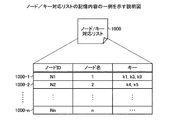•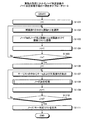•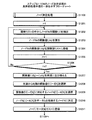•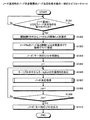•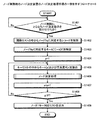•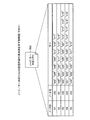•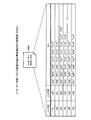## Classifications

• GPHYSICS
• G06COMPUTING; CALCULATING; COUNTING
• G06FELECTRIC DIGITAL DATA PROCESSING
• G06F16/00Information retrieval; Database structures therefor; File system structures therefor
• G06F16/10File systems; File servers
• G06F16/18File system types
• G06F16/182Distributed file systems
• G06F16/1824Distributed file systems implemented using Network-attached Storage [NAS] architecture
• GPHYSICS
• G06COMPUTING; CALCULATING; COUNTING
• G06FELECTRIC DIGITAL DATA PROCESSING
• G06F16/00Information retrieval; Database structures therefor; File system structures therefor
• G06F16/10File systems; File servers
• G06F16/13File access structures, e.g. distributed indices
• G06F16/134Distributed indices
• GPHYSICS
• G06COMPUTING; CALCULATING; COUNTING
• G06FELECTRIC DIGITAL DATA PROCESSING
• G06F16/00Information retrieval; Database structures therefor; File system structures therefor
• G06F16/10File systems; File servers
• G06F16/13File access structures, e.g. distributed indices
• G06F16/137Hash-based
• GPHYSICS
• G06COMPUTING; CALCULATING; COUNTING
• G06FELECTRIC DIGITAL DATA PROCESSING
• G06F16/00Information retrieval; Database structures therefor; File system structures therefor
• G06F16/20Information retrieval; Database structures therefor; File system structures therefor of structured data, e.g. relational data
• G06F16/22Indexing; Data structures therefor; Storage structures
• G06F16/2228Indexing structures
• G06F16/2255Hash tables
• GPHYSICS
• G06COMPUTING; CALCULATING; COUNTING
• G06FELECTRIC DIGITAL DATA PROCESSING
• G06F16/00Information retrieval; Database structures therefor; File system structures therefor
• G06F16/20Information retrieval; Database structures therefor; File system structures therefor of structured data, e.g. relational data
• G06F16/27Replication, distribution or synchronisation of data between databases or within a distributed database system; Distributed database system architectures therefor

## Abstract

PROBLEM TO BE SOLVED: To reduce data movement among nodes upon increasing/decreasing node numbers.SOLUTION: A node determination apparatus 101 associates respective different functions f_1() to f_3() for every node N1 to N3. The node determination apparatus 101 substitutes a key k of data D for the functions f_1(), f_2(), and f_3() for every node N1 to N3 respectively to calculate function values f_1(k), f_2(k), and f_3(k) for every node N1 to N3. The node determination apparatus 101 determines a node N for storing the data D based on the magnitude relation among the function values f_1(k), f_2(k), and f_3(k) for every node N1 to N3.

## Description

The present invention relates to a node determination program, a node determination device, and a node determination method for determining a node that stores data.

Some types of distributed data stores have different nodes for storing data specified from the keys for each data key. In the distributed data store, data with the same key may be redundantly stored in a plurality of nodes from the viewpoint of fault tolerance. Furthermore, the number of nodes in the distributed data store may be dynamically increased or decreased without stopping the service as the data store.

In such a distributed data store, for each data key, a node for storing data specified from the key is determined from a plurality of nodes (so-called key space division problem). As a conventional method, for example, one hash function h () is determined, and the hash value h (k) obtained by giving the data key “k” to the hash function h () is divided by the number of nodes. There is a method for determining a node for storing data.

In addition, as a related prior art, for example, a node that holds data divided in a data maintenance device does not have management information such as arrangement information of divided data, and acquires and distributes divided data held by other nodes. (For example, refer to Patent Document 1 below).

JP 2007-73003 A

However, the above-described conventional technique has a problem in that when the number of nodes in the distributed data store is increased or decreased, the storage destination of a lot of data may change, and the data movement between the nodes increases. For example, in the conventional method described above, when the number of nodes in the distributed data store is increased from 10 to 11, the storage destination of 10/11 data of all data is changed.

An object of the present invention is to provide a node determination program, a node determination device, and a node determination method capable of reducing data movement between nodes when the number of nodes increases or decreases, in order to solve the above-described problems caused by the prior art. To do.

In order to solve the above-described problems and achieve the object, the node determination program, the node determination apparatus, and the node determination method are different functions for each node included in a plurality of nodes, and the domain of the function stores data. A function that includes a key for uniquely specifying the function and a function in which the magnitude relation of the value of the function is defined is associated for each node, and the key is assigned to the associated function for each node. Thus, the function value for each node is calculated, the node for storing the data is determined based on the calculated magnitude relationship of the function value for each node, and the determined determination result is output.

According to the node determination program, the node determination device, and the node determination method, there is an effect that data movement between nodes can be reduced when the number of nodes increases or decreases.

It is explanatory drawing which shows one Example of the node determination process of the node determination apparatus concerning embodiment. It is explanatory drawing which shows an example of the data movement at the time of a node addition. It is explanatory drawing which shows an example of the data movement at the time of node deletion. It is explanatory drawing which shows an example of the distribution system concerning embodiment. It is a block diagram which shows an example of the hardware constitutions of the computer used for embodiment. It is a block diagram which shows the functional structure of the node determination apparatus concerning embodiment. It is explanatory drawing which shows an example of the memory content of a key list. It is explanatory drawing which shows an example of the memory content of a function list. It is explanatory drawing which shows an example of the memory content of a function value list. It is explanatory drawing which shows an example of the memory content of a node / key corresponding list. It is a flowchart which shows an example of the node determination processing procedure of the node determination apparatus concerning embodiment. It is a flowchart which shows an example of the specific process sequence of the node determination process of step S1108. It is a flowchart which shows an example of the node determination processing procedure of the node determination apparatus at the time of node addition. It is a flowchart which shows an example of the node determination processing procedure of the node determination apparatus at the time of node deletion. It is explanatory drawing (the 1) which shows the specific example of the memory content of a node / key correspondence list. It is explanatory drawing (the 2) which shows the specific example of the memory content of a node / key correspondence list.

Exemplary embodiments of a node determination program, a node determination apparatus, and a node determination method according to the present invention will be explained below in detail with reference to the accompanying drawings.

(One Example of Node Determination Process)
FIG. 1 is an explanatory diagram of an example of the node determination process of the node determination device according to the embodiment. Here, a case where the node N for storing the data D is determined from the nodes N1 to N3 in the distributed data store will be described as an example.

Here, the distributed data store is a system that distributes and stores data groups in a plurality of nodes (here, nodes N1 to N3). In the distributed data store, the data D and the key k are paired, and the data D can be referred to by specifying the key k. The key k of the data D is information for uniquely specifying the data D.

In FIG. 1, first, the node determination apparatus 101 associates the node N1 with the function f_1 (). Further, the node determination apparatus 101 associates the node N2 with the function f_2 (). Further, the node determination apparatus 101 associates the node N3 with the function f_3 (). Here, the functions f_1 (), f_2 (), and f_3 () are different functions, and the domain of the function includes the key k of the data D, and the value of the function (function value) in the function range. This is a function for which the magnitude relation of is defined.

Next, the node determination apparatus 101 substitutes the key k of the data D for each of the functions f_1 (), f_2 (), and f_3 () for each of the nodes N1 to N3, so that the function value f_1 (for each of the nodes N1 to N3). k), f_2 (k), and f_3 (k) are calculated.

Then, the node determination device 101 determines the node N that stores the data D based on the magnitude relationship between the function values f_1 (k), f_2 (k), and f_3 (k) for each of the nodes N1 to N3. Here, as an example, the node determining apparatus 101 stores a node N3 corresponding to the minimum function value f_3 (k) among the function values f_1 (k), f_2 (k), and f_3 (k), and a node that stores the data D N is determined.

According to the embodiment described above, data movement between nodes when the number of nodes in the distributed data store is increased or decreased can be reduced. Specifically, even when the number of nodes in the distributed data store changes, the magnitude relationship between the function values of the nodes N1 to N3 does not change, so that occurrence of data movement between the nodes can be suppressed.

More specifically, for example, when the number of nodes in the distributed data store increases, the data D does not move unless the function value of the newly added node N is minimized. On the other hand, when the number of nodes in the distributed data store decreases, the data D does not move unless the node N3 is deleted. Hereinafter, data movement between nodes when the number of nodes is increased or decreased will be described with reference to FIGS. 2 and 3.

(Example of data movement when adding a node)
FIG. 2 is an explanatory diagram showing an example of data movement when a node is added. Here, a case where a new node N4 is added to the distributed data store after data D is stored in the node N3 will be described. Here, even when the node N4 is added, the magnitude relationship between the function values f_1 (k), f_2 (k), and f_3 (k) of the nodes N1 to N3 does not change.

Therefore, as a result of calculating the function value f_4 (k) for the function f_4 () of the new node N4, the minimum function value among the function values f_1 (k) to f_4 (k) is the following pattern 1 or pattern Either one. Pattern 1 is a case where the function value f_3 (k) of the node N3 is still the minimum. Pattern 2 is a case where the function value f_4 (k) of the node N4 is minimized.

Here, in the case of the pattern 1, the movement of the data D does not occur. On the other hand, in the case of pattern 2, the node N4 is determined as the node N for storing the data D, and the data D stored in the node N3 moves to the node N4. That is, when the node is added, the data D does not move unless the function value f_4 (k) of the node N4 is minimized, so that the data movement between the nodes can be suppressed.

(Example of data movement when deleting a node)
FIG. 3 is an explanatory diagram showing an example of data movement when a node is deleted. Here, the case where the node N3 in the distributed data store is deleted after the data D is stored in the node N3 (pattern 3) and the case where the node N2 is deleted (pattern 4) will be described.

In the case of pattern 3, since the node N3 is deleted, movement of the data D stored in the node N3 occurs. However, even when the node N3 is deleted, the magnitude relationship between the function values f_1 (k) and f_2 (k) of the remaining nodes N1 and N2 does not change. Therefore, the data D stored in the node N3 moves to the node N1 having the smallest function value next to the node N3. On the other hand, in the case of the pattern 4, the movement of the data D does not occur. That is, when the node is deleted, the data D does not move unless the node N3 storing the data D is deleted, so that the data movement between the nodes can be suppressed.

(Example of distributed system 400)
FIG. 4 is an explanatory diagram of an example of the distributed system according to the embodiment. In FIG. 4, the distributed system 400 includes a node determination device 101, nodes N1 to Nn, and a client device C. In the distributed system 400, the node determination device 101, the nodes N1 to Nn, and the client device C are connected to be communicable with each other via a network 410 such as the Internet, a LAN (Local Area Network), and a WAN (Wide Area Network). Has been.

Here, the nodes N1 to Nn are servers such as a file server and a database server, for example. The client device C is, for example, a computer that receives a data store service. The client device C can refer to the data D stored in any one of the nodes N in the distributed data store DS using the key k of the data D.

In the following description, an arbitrary node N among the plurality of nodes N1 to Nn is expressed as “node Ni” (i = 1, 2,..., N). A data group to be stored is represented as “data D1 to Dm”, and arbitrary data D among the data D1 to Dm is represented as “data Dj” (j = 1, 2,..., M). A key for uniquely specifying the data Dj is denoted as “key kj”.

(Computer hardware configuration)
Next, a hardware configuration of a computer (node determination device 101, nodes N1 to Nn, client device C) used in the embodiment will be described.

FIG. 5 is a block diagram illustrating an example of a hardware configuration of a computer used in the embodiment. In FIG. 5, the computer includes a CPU (Central Processing Unit) 501, a ROM (Read-Only Memory) 502, a RAM (Random Access Memory) 503, a magnetic disk drive 504, a magnetic disk 505, and an optical disk drive 506. , An optical disc 507, a display 508, an I / F (Interface) 509, a keyboard 510, and a mouse 511. Each component is connected by a bus 500.

Here, the CPU 501 governs overall control of the computer. The ROM 502 stores a program such as a boot program. The RAM 503 is used as a work area for the CPU 501. The magnetic disk drive 504 controls reading / writing of data with respect to the magnetic disk 505 according to the control of the CPU 501. The magnetic disk 505 stores data written under the control of the magnetic disk drive 504.

The optical disk drive 506 controls the reading / writing of the data with respect to the optical disk 507 according to control of CPU501. The optical disk 507 stores data written under the control of the optical disk drive 506, and causes the computer to read data stored on the optical disk 507.

The display 508 displays data such as a document, an image, and function information as well as a cursor, an icon, or a tool box. As the display 508, for example, a CRT, a TFT liquid crystal display, a plasma display, or the like can be adopted.

The I / F 509 is connected to a network 410 such as a LAN, a WAN, or the Internet through a communication line, and is connected to another device via the network 410. The I / F 509 manages an internal interface with the network 410 and controls data input / output from an external device. For example, a modem or a LAN adapter may be employed as the I / F 509.

The keyboard 510 includes keys for inputting characters, numbers, various instructions, and the like, and inputs data. Moreover, a touch panel type input pad or a numeric keypad may be used. The mouse 511 moves the cursor, selects a range, moves the window, changes the size, and the like. A trackball or a joystick may be used as long as they have the same function as a pointing device.

(Functional configuration of the node determination apparatus 101)
Next, a functional configuration of the node determination apparatus 101 according to the embodiment will be described. FIG. 6 is a block diagram of a functional configuration of the node determination device according to the embodiment. In FIG. 6, the node determination apparatus 101 includes a reception unit 601, an association unit 602, a calculation unit 603, a determination unit 604, a rearrangement unit 605, a selection unit 606, and an output unit 607. is there. Each functional unit (accepting unit 601 to output unit 607) causes the CPU 501 to execute a program stored in a storage device such as the ROM 502, the RAM 503, the magnetic disk 505, and the optical disk 507 illustrated in FIG. The function is realized by the I / F 509. Further, the processing results of the respective function units (reception unit 601 to output unit 607) are stored in a storage device such as the RAM 503, the magnetic disk 505, and the optical disk 507, unless otherwise specified.

The accepting unit 601 has a function of accepting key information related to data Dj to be stored. Here, the key information includes, for example, the data name of the data Dj to be stored, the key kj, and the redundancy Rj. The data Dj is, for example, information in units of folders, files, and records. The key kj is, for example, a character string such as a file path name or a primary key of a record in the database. The redundancy Rj is the number of nodes when the data Dj of the same key kj is redundantly stored in a plurality of nodes Ni from the viewpoint of fault tolerance.

Specifically, for example, the receiving unit 601 receives key information by a user operation input using the keyboard 510 and the mouse 511 illustrated in FIG. Further, the reception unit 601 may receive key information from the client apparatus C via the network 410. The received key information is stored in, for example, the key list 700 shown in FIG.

FIG. 7 is an explanatory diagram showing an example of the stored contents of the key list. In FIG. 7, a key list 700 has fields of data name, key, and redundancy, and key information 700-1 to 700-m is stored as records by setting information in each field. Here, the data name is the name of the data Dj. The key is information for uniquely specifying the data Dj. The redundancy is the number of nodes when data Dj is redundantly stored in a plurality of nodes Ni.

The stored contents of the key list 700 are updated each time, for example, when key information is received or when data Dj is deleted from the distributed data store DS. The function of the key list 700 is realized by a storage device such as the ROM 502, the RAM 503, the magnetic disk 505, and the optical disk 507, for example.

Returning to the description of FIG. 6, the receiving unit 601 has a function of receiving a node determination instruction that accompanies an increase or decrease in the number of nodes in the distributed data store DS. Here, the node determination instruction is an instruction to redetermine the node Ni as the storage destination of the data Dj as the number of nodes increases or decreases.

Specifically, the node determination instruction accompanying the node addition includes, for example, the node name of the node Ni added to the distributed data store DS. The addition of a new node Ni is performed for the purpose of improving the performance of the distributed system 400, for example. Further, the node determination instruction accompanying the node deletion includes, for example, the node name of the node Ni to be deleted from the distributed data store DS. Note that the deletion of the node Ni is performed, for example, when the node Ni fails.

Specifically, for example, the reception unit 601 receives a node determination instruction by a user operation input using the keyboard 510 or the mouse 511. Further, the receiving unit 601 may receive a node determination instruction from the node Ni or the client device C via the network 410.

The associating unit 602 has a function of associating a function for each node Ni. Hereinafter, the function of the node Ni is expressed as “function f_i ()”. Here, the function f_i () is a function that is different for each node Ni, and the domain of the function f_i () includes the key kj of the data Dj, and the magnitude relation of the function value is defined in the range. In other words, the function f_i () is a function whose domain includes a range of values that can be taken by the key kj, and in which the magnitude relationship between the function values can be compared.

Specifically, for example, the associating unit 602 selects an arbitrary function as the function f_i () from the function group F prepared in advance, and associates the node Ni with the function f_i (). The association result is stored, for example, in a function list 800 shown in FIG. The function group F is a set of functions corresponding to at least n nodes, and is stored in a storage device such as the ROM 502, the RAM 503, the magnetic disk 505, and the optical disk 507.

Alternatively, the associating unit 602 may prepare one function f () that takes two arguments, and define the function f_i () as, for example, the following equation (1). Here, i is a node name of the node Ni, and kj is a key of the data Dj. The function f () taking two arguments is stored in a storage device such as the ROM 502, the RAM 503, the magnetic disk 505, and the optical disk 507, for example.

f_i () == f (i, kj) (1)

As the function f_i (), any function can be used as long as it satisfies the above-mentioned domain and range conditions. Specifically, for example, a function that can obtain a fixed-length random number by giving a key as an argument may be used as the function f_i (). More specifically, for example, a hash function such as sha1 (secure hash algorithm 1) may be used as the function f_i (). sha1 is a function having the property that the output is greatly different if the input is slightly different. For example, when the key kj and the node name “i” are both integers, a function “f_i () = kj + i” for adding the node name “i” to the key kj is used as the function f_i (). Also good.

Further, as the functions f_1 () to f_n () of the nodes N1 to Nn, functions whose frequency distributions of function values are sufficiently equal and independent from each other may be used. Specifically, for example, the associating unit 602 may define the function f_i () as shown in the following formula (2) using a hash function such as sha1. However, “concatenate (i, kj)” is a function that concatenates the node name “i” of the node Ni and the key kj of the data Dj as a character string.

f_i () == sha1 (concatenate (i, kj)) (2)

FIG. 8 is an explanatory diagram of an example of the contents stored in the function list. In FIG. 8, a function list 800 has fields of node ID, node name, and function, and function information 800-1 to 800-n is stored as records by setting information in each field.

The node ID is an identifier of the node Ni used for explanation in this specification. The node name is the name of the node Ni. The function is a function f_i () associated with the node Ni. The function list 800 implements its functions by a storage device such as the ROM 502, the RAM 503, the magnetic disk 505, and the optical disk 507, for example.

Returning to the description of FIG. 6, the calculation unit 603 assigns the key kj to the function f_i () for each associated node Ni, thereby obtaining the value of the function f_i () for each node Ni (hereinafter, “function value f_i”). (K) ")) is calculated. Specifically, for example, the calculation unit 603 calculates the function value f_i (k) for each node Ni by giving the key k to the function f_i () as an argument.

Further, when the above equation (1) is used as the function f_i (), the calculation unit 603 gives the node name “i” of the node Ni and the key kj to the equation (1) as arguments, so that the function for each node Ni is obtained. The value f_i (k) is calculated. The calculated result is stored in, for example, a function value list 900 shown in FIG.

FIG. 9 is an explanatory diagram of an example of the contents stored in the function value list. In FIG. 9, a function value list 900 has fields of node ID, node name, and function value, and by setting information in each field, function value information 900-1 to 900-n is stored as a record. Yes. The function value list 900 realizes its function by a storage device such as the ROM 502, the RAM 503, the magnetic disk 505, and the optical disk 507, for example.

Returning to the description of FIG. 6, the determination unit 604 has a function of determining the node Ni that stores the data Dj based on the magnitude relationship of the calculated function value f_i (kj) for each node Ni. Specifically, for example, the determination unit 604 stores data Dj for the node Ni corresponding to the minimum (or maximum) function value f_i (kj) among the function values f_1 (kj) to f_n (kj). Node N is determined.

The rearrangement unit 605 has a function of rearranging the function value f_i (kj) for each node Ni based on the calculated magnitude relationship of the function value f_i (kj) for each node Ni. Specifically, for example, the rearrangement unit 605 rearranges the function values f_1 (kj) to f_n (kj) of the nodes N1 to Nn in ascending order (or descending order).

The selection unit 606 has a function of selecting a predetermined number of nodes Ni from the nodes N1 to Nn according to the order of the function values f_i (kj) for each rearranged node Ni. Here, the predetermined number is, for example, the redundancy Rj of the data Dj. In the following description, the function values f_1 (kj) to f_n (kj) of the rearranged nodes N1 to Nn are expressed as “function values f  to f [n]”.

Specifically, for example, first, the selection unit 606 performs Rj function values f  to f [Rj from the beginning (or the tail) of the rearranged function values f  to f [n]. ] Is selected. Then, the selection unit 606 refers to the function value list 900 to identify and select Rj nodes corresponding to the selected function values f  to f [Rj].

In the following description, the selected Rj nodes are denoted as “nodes N  to N [Rj]”. When the redundancy Rj is “Rj = 1”, the selection unit 606 selects nodes Ni in a specific order from the head (or tail) of the rearranged function values f  to f [n]. You may decide to choose.

The determination unit 604 may determine the selected predetermined number of nodes Ni as the nodes N that store the data Dj. Specifically, for example, the determination unit 604 determines the selected Rj nodes N  to N [Rj] as the nodes N that store the data Dj. The determined determination result is stored in the node / key correspondence list 1000 shown in FIG.

FIG. 10 is an explanatory diagram of an example of the contents stored in the node / key correspondence list. In FIG. 10, the node / key correspondence list 1000 has fields of node ID, node name, and key. By setting information in each field, correspondence information 1000-1 to 1000-n for each of the nodes N1 to Nn is stored as a record.

As an example, when the node N1 is determined as the node N storing the data D1, D3, D9, the keys k1, k3, k9 of the data D1, D3, D9 are set in the “key” field of the correspondence information 1000-1. Is done. When the node N2 is determined as the node N that stores the data D4 and D5, the keys k4 and k5 of the data D4 and D5 are set in the “key” field of the correspondence information 1000-2. The key kj of each data Dj can be specified by referring to the key list 700, for example.

Returning to the description of FIG. 6, the output unit 607 has a function of outputting the determined determination result. Specifically, for example, the output unit 607 outputs the node / key correspondence list 1000 shown in FIG. Examples of the output format include display on the display 508, print output to a printer (not shown), and transmission to an external device by the I / F 509. Alternatively, the data may be stored in a storage area such as the RAM 503, the magnetic disk 505, and the optical disk 507.

More specifically, for example, the output unit 607 may transmit the node / key correspondence list 1000 to an external computer that controls data movement between nodes. In this case, for example, an external computer controls data movement between nodes according to the node / key correspondence list 1000. Further, the output unit 607 may transmit an instruction to move the data Di to the node Ni according to the node / key correspondence list 1000.

In the above description, the case where the node determination device 101 and the node Ni are provided separately has been described, but the present invention is not limited to this. Specifically, for example, the node Ni may include the node determination device 101.

(Node determination processing procedure of the node determination apparatus 101)
Next, a node determination processing procedure of the node determination apparatus 101 according to the embodiment will be described. Here, a case where the node N that is the storage destination of the data D1 to Dm is determined from the nodes N1 to Nn will be described as an example.

FIG. 11 is a flowchart illustrating an example of a node determination processing procedure of the node determination device according to the embodiment. In the flowchart of FIG. 11, first, the associating unit 602 initializes “i” of the node Ni with “i = 1” (step S1101), and selects the function f_i () from the function group F (step S1102). ). Then, the association unit 602 associates the node name of the node Ni with the selected function f_i () and registers it in the function list 800 (step S1103).

Next, the association unit 602 increments “i” of the node Ni (step S1104), and determines whether “i” is greater than “n” (step S1105). If “i” is equal to or less than “n” (step S1105: No), the process returns to step S1102.

On the other hand, when “i” is larger than “n” (step S1105: Yes), the calculation unit 603 initializes “j” of the data Dj with “j = 1” (step S1106). Then, the calculation unit 603 extracts the key kj and the redundancy Rj of the data Dj from the key list 700 (step S1107).

Thereafter, the determination unit 604 executes node determination processing for determining the node N that stores the data Dj (step S1108). The calculation unit 603 increments “j” of the data Dj (step S1109), and determines whether “j” is greater than “m” (step S1110). If “j” is equal to or less than “m” (step S1110: No), the process returns to step S1107.

On the other hand, when “j” is larger than “m” (step S1110: Yes), the output unit 607 outputs the node / key correspondence list 1000 (step S1111), and the series of processing according to this flowchart ends.

Next, a specific processing procedure of the node determination processing in step S1108 shown in FIG. 11 will be described. FIG. 12 is a flowchart illustrating an example of a specific processing procedure of the node determination processing in step S1108.

In the flowchart of FIG. 12, the calculation unit 603 first initializes “i” of the node Ni with “i = 1” (step S1201), and extracts the function f_i () of the node Ni from the function list 800. (Step S1202). Next, the calculation unit 603 substitutes the key kj extracted in step S1107 shown in FIG. 11 for the extracted function f_i () to calculate the function value f_i (kj) of the node Ni (step S1203). .

Then, the calculation unit 603 registers the calculated function value f_i (kj) of the node Ni in the function value list 900 (step S1204). Thereafter, the calculation unit 603 increments “i” of the node Ni (step S1205), and determines whether “i” is greater than “n” (step S1206).

If “i” is equal to or smaller than “n” (step S1206: No), the process returns to step S1202. On the other hand, when “i” is greater than “n” (step S1206: Yes), the reordering unit 605 refers to the function value list 900 to rearrange the function values f_1 (kj) to f_n (kj) in ascending order. (Step S1207).

Thereafter, the selection unit 606 selects Rj function values f  to f [Rj] from the top of the rearranged function values f  to f [n] (step S1208). However, Rj is the redundancy Rj extracted in step S1107 shown in FIG. Next, the selection unit 606 refers to the function value list 900 to select nodes N  to N [Rj] corresponding to the selected Rj function values f  to f [Rj]. (Step S1209).

Thereafter, the determination unit 604 determines the selected nodes N  to N [Rj] as the nodes N that store the data Dj (step S1210). Then, the determination unit 604 registers the determined determination result in the node / key correspondence list 1000 (step S1211), and the process proceeds to step S1109 shown in FIG.

Thereby, the node N that stores the data Dj can be determined from the magnitude relationship of the function value f_i (k) obtained by giving the key kj as an argument to the function f_i () for each node Ni. As a result, data movement between nodes when the number of nodes in the distributed data store DS is increased or decreased can be reduced.

(Node decision processing procedure when adding a node)
Next, the node determination processing procedure of the node determination apparatus 101 when adding a node will be described. Here, a node newly added to the distributed data store DS is represented as “node Nx”. FIG. 13 is a flowchart illustrating an example of a node determination processing procedure of the node determination device when adding a node.

In the flowchart of FIG. 13, first, the receiving unit 601 determines whether or not a node determination instruction accompanying node addition has been received (step S1301). Here, it waits for the reception of the node determination instruction (step S1301: No), and when it is received (step S1301: Yes), the association unit 602 selects the function f_x () of the node Nx from the function group F. (Step S1302).

The associating unit 602 associates the node name of the node Nx with the selected function f_x () and registers them in the function list 800 (step S1303). However, the node name of the node Nx and the function f_x () are registered as function information 800-n at the tail of the function list 800.

Next, the determination unit 604 initializes the node / key correspondence list 1000 (step S1304). Then, the calculation unit 603 initializes “j” of the data Dj with “j = 1” (step S1305), and extracts the key kj and the redundancy Rj of the data Dj from the key list 700 (step S1306). .

Thereafter, the determination unit 604 executes node determination processing for determining the node N that stores the data Dj (step S1307). Then, the calculation unit 603 increments “j” of the data Dj (step S1308), and determines whether “j” is greater than “m” (step S1309).

If “j” is equal to or less than “m” (step S1309: NO), the process returns to step S1306. On the other hand, if “j” is greater than “m” (step S1309: YES), the output unit 607 outputs the node / key correspondence list 1000 (step S1310), and the series of processing according to this flowchart ends.

Thereby, when adding a node to the distributed data store DS, the node N storing the data D1 to Dm can be determined again. Further, when data movement occurs with the addition of the node Nx, the data Dj moves to the newly added node Nx, so that the performance when adding the node can be improved efficiently. Note that the specific processing procedure of the node determination processing in step S1307 is the same as the specific processing procedure of the node determination processing in step S1108 shown in FIG.

(Node determination procedure when deleting a node)
Next, the node determination processing procedure of the node determination apparatus 101 at the time of node deletion will be described. Here, a node to be deleted from the distributed data store DS is denoted as “node Ny”. FIG. 14 is a flowchart illustrating an example of a node determination processing procedure of the node determination device when a node is deleted.

In the flowchart of FIG. 14, first, the receiving unit 601 determines whether a node determination instruction accompanying node deletion has been received (step S1401). Here, after waiting for the reception of the node determination instruction (step S1401: No), and when received (step S1401: Yes), the associating unit 602 deletes the record corresponding to the node Ny from the function list 800 ( Step S1402). The node ID in the function list 800 is reassigned after the record corresponding to the node Ny is deleted.

Next, the determining unit 604 refers to the node / key correspondence list 1000 to identify keys corresponding to the node Ny (in this case, expressed as keys k  to k [P]) (step S1403). . Then, the calculation unit 603 initializes “p” of the data Dp with “p = 1” (step S1404), and sets the key k [p] and the redundancy R [p] of the data Dp from the key list 700. Extract (step S1405).

Thereafter, the determination unit 604 executes node determination processing for determining the node N that stores the data Dp (step S1406). Then, the calculation unit 603 increments “p” of the data Dp (step S1407), and determines whether “p” is larger than “P” (step S1408).

If “p” is equal to or less than “P” (step S1408: NO), the process returns to step S1405. On the other hand, if “p” is greater than “P” (step S1408: YES), the output unit 607 outputs the node / key correspondence list 1000 (step S1409), and the series of processes according to this flowchart ends.

As a result, when deleting a node from the distributed data store DS, the node N storing the data D  to D [P] stored in the node Ny to be deleted can be determined again. Note that the specific process procedure of the node determination process in step S1406 is the same as the specific process procedure of the node determination process in step S1108 shown in FIG.

(Specific example of node decision processing)
Next, a specific example of the node determination process of the node determination apparatus 101 will be described. Here, the following formula (3) is used as the function f_i () of the node Ni. However, i represents the node name of the node Ni, kj represents the key of the data Dj, and (i + kj) represents the combination of the node name and the key as a character string.

f_i () = f (i, kj) = <first 32 bits of sha1 (i + kj)>
... (3)

(I) When n = 5 First, a case where the number n of nodes in the distributed data store DS is 5 (n = 5) and the node N that stores the data D1 to D20 is determined from the nodes N1 to N5 will be described. To do. However, the node names of the nodes N1 to N5 are “n00”, “n01”, “n02”, “n03”, and “n04”, respectively.

Further, the redundancy R1 to R20 of each data D1 to D20 is all “2”. Then, the node determining apparatus 101 uses the nodes corresponding to the first and second function values from the top when the function values of the nodes N1 to N5 are rearranged in ascending order as the storage destination of the data Dj specified from the key kj. N is determined. In the following description, 32 bits of the result (function value) of the function f_i (kj) are expressed using hexadecimal numbers.

Here, assuming that the key k1 of the data D1 is “k00”, the function values of the nodes N1 to N5 are calculated by the node determination device 101 using the above formula (1) and rearranged in ascending order as follows. Become.
f_n01 (“k00”) = 0e2ec04a
f_n04 ("k00") = 115aaafa
f_n02 (“k00”) = 326d28c9
f_n03 (“k00”) = 54895176
f_n00 (“k00”) = 85a25d67

In this case, of the nodes N1 to N5, the node N having the smallest function value is the node N2 (node name: n01), and the node N having the next smallest function value is the node N5 (node name: n04). Therefore, the storage destination of the data D1 specified from the key k1 (“k00”) is the nodes N2 and N5.

Next, assuming that the key k2 of the data D2 is “k01”, the function values of the nodes N1 to N5 are calculated by the node determination device 101 using the above formula (1) and rearranged in ascending order as follows. Become.
f_n01 (“k01”) = ac5a52a0
f_n03 (“k01”) = b623b072
f_n00 (“k01”) = d3008e9c
f_n02 (“k01”) = e0c43847
f_n04 (“k01”) = e1ebf581

In this case, among the nodes N1 to N5, the node N having the smallest function value is the node N2 (node name: n01), and the node N having the next smallest function value is the node N4 (node name: n03). Therefore, the storage destination of the data D2 specified from the key k2 (“k01”) is the nodes N2 and N4.

Similarly, when the keys k3 to k20 of the data D3 to D20 are set to “k02” to “k19” and the storage destinations of the data D3 to D20 are determined, the correspondence between the node name and the key is the node / key shown in FIG. A correspondence list 1500 is obtained.

FIG. 15 is an explanatory diagram (part 1) of a specific example of the contents stored in the node / key correspondence list. In FIG. 15, node names and key correspondences are shown for each of the nodes N1 to N5. Here, when the number of keys associated with each of the nodes N1 to N5 is close to “K × R / n”, it can be said that the data D1 to D20 are sufficiently evenly distributed. Here, K is the total number of keys kj, R is the redundancy of data Dj, and n is the number of nodes.

Here, since “K = 20, R = 2, n = 5”, when the number of keys associated with the nodes N1 to N5 is close to “8”, the data D1 to D20 are sufficiently evenly distributed. It can be said that. In the example shown in FIG. 15, 7 keys are associated with the node N1, 7 keys with the node N2, 10 keys with the node N3, 10 keys with the node N4, and 6 keys with the node N5. Therefore, the data D1 to D20 having redundancy 2 are sufficiently evenly distributed.

(Ii) Case where n = 6 Next, a case where the number n of nodes in the distributed data store DS increases from 5 (n = 5) to 6 (n = 6) will be described. Here, the node name of the newly added node N6 is “n05”.

Similarly to the above, when the key k1 of the data D1 is set to “k00”, the node determination device 101 calculates the function values of the nodes N1 to N6 using the above formula (1) and rearranges them in ascending order as follows. Become.
f_n01 (“k00”) = 0e2ec04a
f_n04 ("k00") = 115aaafa
f_n02 (“k00”) = 326d28c9
f_n03 (“k00”) = 54895176
f_n05 (“k00”) = 5633a21a
f_n00 (“k00”) = 85a25d67

In this case, as in the case where the number of nodes n is 5 (n = 5), the node N having the smallest function value among the nodes N1 to N6 is the node N2 (node name: n01), and the next smallest function value The node N taking the node is the node N5 (node name: n04). Therefore, the storage destination of the data D1 specified from the key k1 (“k00”) does not change.

Next, assuming that the key k2 of the data D2 is “k01”, the function values of the nodes N1 to N6 are calculated by the node determination device 101 using the above formula (1) and rearranged in ascending order as follows. .
f_n05 (“k01”) = 58262a5c
f_n01 (“k01”) = ac5a52a0
f_n03 (“k01”) = b623b072
f_n00 (“k01”) = d3008e9c
f_n02 (“k01”) = e0c43847
f_n04 (“k01”) = e1ebf581

In this case, the node N having the smallest function value among the nodes N1 to N6 is the node N6 (node name: n05), and the node N having the next smallest function value is the node N2 (node name: n01). Accordingly, the storage destination of the data D2 specified from the key k2 (“k01”) changes from the nodes N2 and N4 to the nodes N6 and N2.

Similarly, when the keys k3 to k20 of the data D3 to D20 are set to “k02” to “k19” and the storage destinations of the data D3 to D20 are determined, the correspondence between the node names and the keys is the node / key shown in FIG. A correspondence list 1600 is obtained.

FIG. 16 is an explanatory diagram (part 2) of a specific example of the contents stored in the storage location list. In FIG. 16, the correspondence between the node name and the key is shown for each of the nodes N1 to N6. Here, when the total number of data Dj to move when the number of nodes changes (that is, the total number of keys kj) is sufficiently close to “K × R × 1 / (n + 1)”, the data when the number of nodes changes It can be said that the amount of movement is sufficiently close to the minimum.

Here, since “K = 20, R = 2, n = 5”, if the number of keys associated with each of the nodes N1 to N5 is close to “7”, the data movement amount is sufficiently close to the minimum. I can say that. In the example shown in FIG. 16, as a result of adding the node N6, data D2 and D6 specified from the keys “k01”, “k05”, “k06”, “k07”, “k09”, “k13”, “k19”. , D7, D8, D10, D14, and D20 are changed. Therefore, the amount of data movement when the number of nodes changes is sufficiently close to the minimum.

As described above, according to the node determination device 101 according to the embodiment, a different function f_i () is prepared for each node Ni, and the magnitude of the function value f_i (k) obtained using the key kj of the data Dj as an argument is large. From the relationship, the node N that stores the data Dj can be determined. Thereby, since the magnitude relationship of the function value f_i (k) between the original nodes does not change even if the number of nodes increases or decreases thereafter, the data Dj does not move between nodes other than the node to be added or deleted.

Further, according to the node determination device 101, it is possible to determine a predetermined number of nodes N that store the data Dj according to the order after the function values f_i (k) for each node Ni are rearranged based on the magnitude relationship. it can. As a result, even when the data Dj is redundantly stored in the Rj nodes Ni, the magnitude relationship of the function value f_i (k) between the original nodes does not change even if the number of nodes increases or decreases thereafter. Data Dj does not move between nodes other than the node to be added or deleted.

Further, according to the node determination device 101, it is possible to determine the node N that stores the data Dj by using a pair of the node name and the function f_i () for each node Ni. For this reason, the amount of information for determining the storage destination of the data Dj is reduced as compared with the method of managing the node N storing the data Dj for each key kj and determining the node N storing the data Dj. Can do. Further, when the function f () having two arguments as in the above formula (1) is used, the node N storing the data Dj can be determined using the function f () and the node name for each node Ni. Therefore, the amount of information can be further reduced.

Further, according to the node determination apparatus 101, the functions f_1 () to f_n () have the function value frequency distributions sufficiently equal and use functions independent of each other, so that the data D1 to Dm are evenly distributed to the nodes N1 to Nn. Can be dispersed. Specifically, of the function values f_1 (kj) to f_n (kj), the probability that the arbitrary function value f_i (kj) is minimum (or maximum) is “1 / n”. Similarly, the probability that an arbitrary function value f_i (kj) is i-th smallest (or larger) is also “1 / n”. Therefore, the storage destinations of the data Dj are determined equally, and the data D1 to Dm are sufficiently evenly distributed to the nodes N1 to Nn.

Further, according to the node determination device 101, a plurality of cases in which data Dj is redundantly stored by using functions f_1 () to f_n () that are sufficiently equal in frequency distribution of function values and independent from each other are used. The combinations of the nodes Ni can be varied. Specifically, among the function values f_1 (kj) to f_n (kj), the function value f_y (kj) is the second smallest with respect to the key kj having the minimum (or maximum) function value f_x (kj). The probability of becoming (or larger) is “1 / (n−1)”. Therefore, even when the data Dj is redundantly stored in the plurality of nodes Ni, the storage destination of the data Dj is determined equally, and the combination of the plurality of nodes Ni can be varied. Thereby, for example, when data Dj is redundantly stored in the nodes N1 to N3, it is possible to avoid a situation in which a load is always applied to the nodes N2 and N3 when a failure occurs in the node N1. .

The node determination method described in the present embodiment can be realized by executing a program prepared in advance on a computer such as a personal computer or a workstation. The node determination program is recorded on a computer-readable recording medium such as a hard disk, a flexible disk, a CD-ROM, an MO, and a DVD, and is executed by being read from the recording medium by the computer. The node determination program may be distributed through a network such as the Internet.

DESCRIPTION OF SYMBOLS 101 Node determination apparatus 400 Distributed system 601 Reception part 602 Association part 603 Calculation part 604 Determination part 605 Rearrangement part 606 Selection part 607 Output part 700 Key list 800 Function list 900 Function value list 1000 Node / key corresponding list C Client apparatus N1- Nn node

## Claims (7)

1. A computer that determines a node for storing data from a plurality of nodes.
The function is different for each node included in the plurality of nodes, and the domain of the function includes a key for uniquely identifying the data, and the magnitude relation of the value of the function is defined in the range of the function. Associating the function for each node;
A calculation step of calculating a value of the function for each node by substituting the key into the function for each node associated by the association step;
A determination step of determining a node for storing the data, based on a magnitude relationship of the value of the function for each node calculated by the calculation step;
An output step of outputting the determination result determined by the determination step;
A node determination program characterized by causing
2. A rearrangement step of rearranging the value of the function for each node based on the magnitude relationship of the value of the function for each node calculated by the calculation step;
A selection step of selecting a predetermined number of nodes from the plurality of nodes according to the order of the function values for each of the nodes rearranged by the rearrangement step;
The node determining program according to claim 1, wherein the determining step determines the predetermined number of nodes selected by the selecting step as nodes storing the data.
3.   2. When adding a new node to the plurality of nodes, the computer causes the computer to execute the association step, the calculation step, and the determination step for each piece of data stored in the plurality of nodes. The node determination program according to 1 or 2.
4.   4. When deleting a node from the plurality of nodes, the computer causes the computer to execute the association step, the calculation step, and the determination step for each piece of data stored in the node. The node determination program according to any one of the above.
5.   The node determination program according to claim 1, wherein the function is a function that obtains a fixed-length random number by giving the key as an argument.
6. In a node determination device for determining a node for storing data from a plurality of nodes
The function is different for each node included in the plurality of nodes, and the domain of the function includes a key for uniquely identifying the data, and the magnitude relation of the value of the function is defined in the range of the function. Associating means for associating the function for each node;
Calculating means for calculating a value of the function for each node by substituting the key into the function for each of the nodes associated by the associating means;
Determining means for determining a node for storing the data, based on a magnitude relation of the value of the function for each node calculated by the calculating means;
Output means for outputting the determination result determined by the determination means;
A node determination device comprising:
7. A computer that determines a node for storing data from a plurality of nodes.
The function is different for each node included in the plurality of nodes, and the domain of the function includes a key for uniquely identifying the data, and the magnitude relation of the value of the function is defined in the range of the function. Associating the function for each node;
A calculation step of calculating a value of the function for each node by substituting the key into the function for each node associated by the association step;
A determination step of determining a node for storing the data, based on a magnitude relationship of the value of the function for each node calculated by the calculation step;
An output step of outputting the determination result determined by the determination step;
The node determination method characterized by performing.
JP2010154332A 2010-07-06 2010-07-06 Node determination program, node determination apparatus, and node determination method Withdrawn JP2012018487A (en)

## Priority Applications (1)

Application Number Priority Date Filing Date Title
JP2010154332A JP2012018487A (en) 2010-07-06 2010-07-06 Node determination program, node determination apparatus, and node determination method

## Applications Claiming Priority (2)

Application Number Priority Date Filing Date Title
JP2010154332A JP2012018487A (en) 2010-07-06 2010-07-06 Node determination program, node determination apparatus, and node determination method
US13/157,799 US20120011171A1 (en) 2010-07-06 2011-06-10 Node determination apparatus and node determination method

## Publications (1)

Publication Number Publication Date
JP2012018487A true JP2012018487A (en) 2012-01-26

# Family

## Family Applications (1)

Application Number Title Priority Date Filing Date
JP2010154332A Withdrawn JP2012018487A (en) 2010-07-06 2010-07-06 Node determination program, node determination apparatus, and node determination method

## Country Status (2)

US (1) US20120011171A1 (en)
JP (1) JP2012018487A (en)

## Families Citing this family (2)

* Cited by examiner, † Cited by third party
Publication number Priority date Publication date Assignee Title
US9201890B2 (en) * 2010-10-04 2015-12-01 Dell Products L.P. Storage optimization manager
JP2015132972A (en) * 2014-01-14 2015-07-23 株式会社野村総合研究所 Data relocation system

## Family Cites Families (10)

* Cited by examiner, † Cited by third party
Publication number Priority date Publication date Assignee Title
CA2317081C (en) * 2000-08-28 2004-06-01 Ibm Canada Limited-Ibm Canada Limitee Estimation of column cardinality in a partitioned relational database
AU2003209790A1 (en) * 2003-03-07 2004-09-28 Nokia Corporation A method and a device for frequency counting
ES2294509T3 (en) * 2003-06-03 2008-04-01 NOKIA SIEMENS NETWORKS GMBH &amp; CO. KG Procedure for distributing traffic by hash codes according to a distribution of setting traffic in a network oriented to packages with multivity route.
US20050060535A1 (en) * 2003-09-17 2005-03-17 Bartas John Alexander Methods and apparatus for monitoring local network traffic on local network segments and resolving detected security and network management problems occurring on those segments
US8266237B2 (en) * 2005-04-20 2012-09-11 Microsoft Corporation Systems and methods for providing distributed, decentralized data storage and retrieval
US7925624B2 (en) * 2006-03-31 2011-04-12 Amazon Technologies, Inc. System and method for providing high availability data
US7788220B1 (en) * 2007-12-31 2010-08-31 Emc Corporation Storage of data with composite hashes in backup systems
JP5369502B2 (en) * 2008-06-04 2013-12-18 株式会社リコー Device, management device, device management system, and program
US8212695B2 (en) * 2009-02-05 2012-07-03 Polytechnic Institute Of New York University Generating a log-log hash-based hierarchical data structure associated with a plurality of known arbitrary-length bit strings used for detecting whether an arbitrary-length bit string input matches one of a plurality of known arbitrary-length bit strings
EP2348449A3 (en) * 2009-12-18 2013-07-10 CompuGroup Medical AG A computer implemented method for performing cloud computing on data being stored pseudonymously in a database

## Also Published As

Publication number Publication date
US20120011171A1 (en) 2012-01-12

## Similar Documents

Publication Publication Date Title
JP2502469B2 (en) Character de - compressed de compressing the data - how to provide a static dictionary structure for deploying data and means
JP5082310B2 (en) Data migration apparatus and program
RU2360288C2 (en) Files containing mixed raster
Afrati et al. Fuzzy joins using mapreduce
WO2012056731A1 (en) Resource management server, resource management method and storage medium in which resource management program is stored
US7927030B2 (en) Printing control method and apparatus
JP4782490B2 (en) Data set dividing program, data set dividing apparatus, and data set dividing method
US5926824A (en) System and method for retrieving a document by inputting a desired attribute and the number of areas in which the attribute occurs as a retrieval condition
US8898168B2 (en) Information searching apparatus, information searching method, and computer product
Rottenstreich et al. The variable-increment counting Bloom filter
WO2011033582A1 (en) Storage system for eliminating duplicated data
JP2009524883A (en) Presenting digital content to the network
CN100561480C (en) Structured document management system and method of managing indexes in the same system
JPH1196145A (en) Storage control system for document image data
US7243304B2 (en) Apparatus and method for creating a map of a real name word to an anonymous word for an electronic document
US8456477B2 (en) Information processing apparatus, information processing method and program for generating and displaying network structures
CN1239253A (en) Method for adapting user interface elements based on historical usage
US7505172B2 (en) Method and systems for processing print jobs
EP2405358A1 (en) Integral double exclusion system, data storage device, and server device
Wu Notes on design and implementation of compressed bit vectors
US8214388B2 (en) System and method for adding a storage server in a distributed column chunk data store
JP2002244898A (en) Database managing program and database system
KR20010077983A (en) Method and means for classifying data packets
US20100119151A1 (en) System and method for binary persistence format for a recognition result lattice
JP2007122302A (en) Information retrieval system, index management method, and program

## Legal Events

Date Code Title Description
A621 Written request for application examination

Free format text: JAPANESE INTERMEDIATE CODE: A621

Effective date: 20130507

A761 Written withdrawal of application

Free format text: JAPANESE INTERMEDIATE CODE: A761

Effective date: 20130723